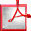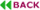TruncApp: A Truncation-based Approximate Divider for Energy Efficient DSP Applications

Shaghayegh Vahdat1,a, Mehdi Kamal1,b, Ali Afzali-Kusha1,c, Massoud Pedram2 and Zainalabedin Navabi1,d
1School of Electrical and Computer Engineering, University of Tehran, Iran.
avahdat_s@ut.ac.ir
bm.kamal@ut.ac.ir
cafzali@ut.ac.ir
dnavabi@ut.ac.ir
2Department of Electrical Engineering, University of Southern California, USA.
pedram@usc.edu

ABSTRACT

In this paper, we present a high speed yet energy efficient approximate divider where the division operation is performed by multiplying the dividend by the inverse of the divisor. In this structure, truncated value of the dividend is multiplied exactly (approximately) by the approximate inverse value of divisor. To assess the efficacy of the proposed divider, its design parameters are extracted and compared to those of a number of prior art dividers in a 45nm CMOS technology. Results reveal that this structure provides 66% and 52% improvements in the area and energy consumption, respectively, compared to the most advanced prior art approximate divider. In addition, delay and energy consumption of the division operation are reduced about 94.4% and 99.93%, respectively, compared to those of an exact SRT radix-4 divider. Finally, the efficacy of the proposed divider in image processing application is studied.

Keywords: Approximate divider, Truncating, Low power, Energy efficient.Full Text (PDF)